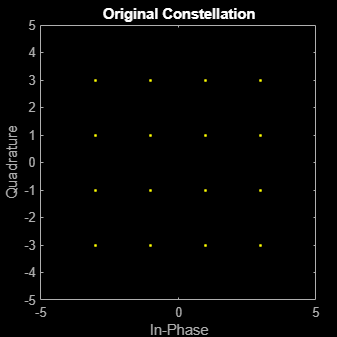# comm.PhaseFrequencyOffset

Apply phase and frequency offsets to input signal

## Description

The `PhaseFrequencyOffset` object applies phase and frequency offsets to an incoming signal.

To apply phase and frequency offsets to the input signal:

1. Create the `comm.PhaseFrequencyOffset` object and set its properties.

2. Call the object with arguments, as if it were a function.

## Creation

### Syntax

``pfo = comm.PhaseFrequencyOffset``
``pfo = comm.PhaseFrequencyOffset(Name=Value)``

### Description

````pfo = comm.PhaseFrequencyOffset` creates a phase and frequency offset System object™. This object applies phase and frequency offsets to an input signal.```

example

````pfo = comm.PhaseFrequencyOffset(Name=Value)` creates a phase and frequency offset object with each specified property set to the specified value. For example, `SampleRate=20` sets a sample rate of 20 Hz. You can specify additional name-value pair arguments in any order as (`Name1`=`Value1`,...,`NameN`=`ValueN`).```

## Properties

expand all

Unless otherwise indicated, properties are nontunable, which means you cannot change their values after calling the object. Objects lock when you call them, and the `release` function unlocks them.

If a property is tunable, you can change its value at any time.

Phase offset in degrees, specified as numeric scalar, an M-by-1 or 1-by-N numeric vector, or an M-by-N numeric matrix. For more information, see Interdependent Property-Input Dimensions.

Tunable: Yes

Data Types: `double`

Frequency offset source, specified as one of these values

• `"Property"` — Specify the frequency offset using the `FrequencyOffset` property.

• `"Input port"` — Specify the frequency offset in the `fOffset` input argument.

Frequency offset in Hz, specified as a numeric scalar, a numeric row or column vector, or a numeric matrix.

Tunable: Yes

#### Dependency

To enable this property, set the `FrequencyOffsetSource` property to `"Property"`.

Data Types: `double`

Sample rate of the input signal in Hz, specified as a positive scalar.

Data Types: `double`

## Usage

### Syntax

``y = pfo(x)``
``y = pfo(x,fOffset)``

### Description

example

````y = pfo(x)` applies phase and frequency offsets to input signal `x`. To use this syntax, set the `FrequencyOffsetSource` to `"Property"`.```
````y = pfo(x,fOffset)` specifies the frequency offset to apply to `x`. To use this syntax, set the `FrequencyOffsetSource` to `"Input port"`.```

### Input Arguments

expand all

Input signal, specified as a numeric scalar, a numeric row or column vector, or a numeric matrix. For more information, see Interdependent Property-Input Dimensions.

Data Types: `single` | `double`

Frequency offset in Hz, specified as a numeric scalar, a numeric row or column vector, or a numeric matrix. For more information, see the description of the `FrequencyOffset` property and Interdependent Property-Input Dimensions.

#### Dependency

To enable this input, set the `FrequencyOffsetSource` property to ```"Input port"```.

Data Types: `single` | `double`

### Output Arguments

expand all

Output signal, returned with the same dimensions and data type as `x`.

Data Types: `single` | `double`

## Object Functions

To use an object function, specify the System object as the first input argument. For example, to release system resources of a System object named `obj`, use this syntax:

`release(obj)`

expand all

 `step` Run System object algorithm `release` Release resources and allow changes to System object property values and input characteristics `reset` Reset internal states of System object

## Examples

collapse all

Introduce a phase offset to a 16-QAM signal and view its effect on the constellation.

Create a phase frequency offset System object™, setting the phase offset to 30 degrees.

`pfo = comm.PhaseFrequencyOffset(PhaseOffset=30);`

Generate random symbols and apply 16-QAM modulation.

```M = 16; data = (0:M-1)'; x = qammod(data,M);```

Plot the 16-QAM constellation.

```scatterplot(x); title("Original Constellation") xlim([-5 5]) ylim([-5 5])```Introduce a phase offset and plot the offset constellation.

```y = pfo(x); scatterplot(y); title("Constellation After Phase Offset") xlim([-5 5]) ylim([-5 5])```expand all

## Algorithms

If the input signal is u(t), then the output signal is

`$y\left(t\right)=u\left(t\right)\cdot \left(\mathrm{cos}\left(2\pi {\int }_{0}^{t}f\left(\tau \right)d\tau +\phi \left(t\right)\right)+j\mathrm{sin}\left(2\pi {\int }_{0}^{t}f\left(\tau \right)d\tau +\phi \left(t\right)\right)\right)\text{,}$`

where f(t) is the frequency offset, and φ(t) is the phase offset.

The discrete-time output is given by

where i > 0, and Δt is the sample time.

## Version History

Introduced in R2012a